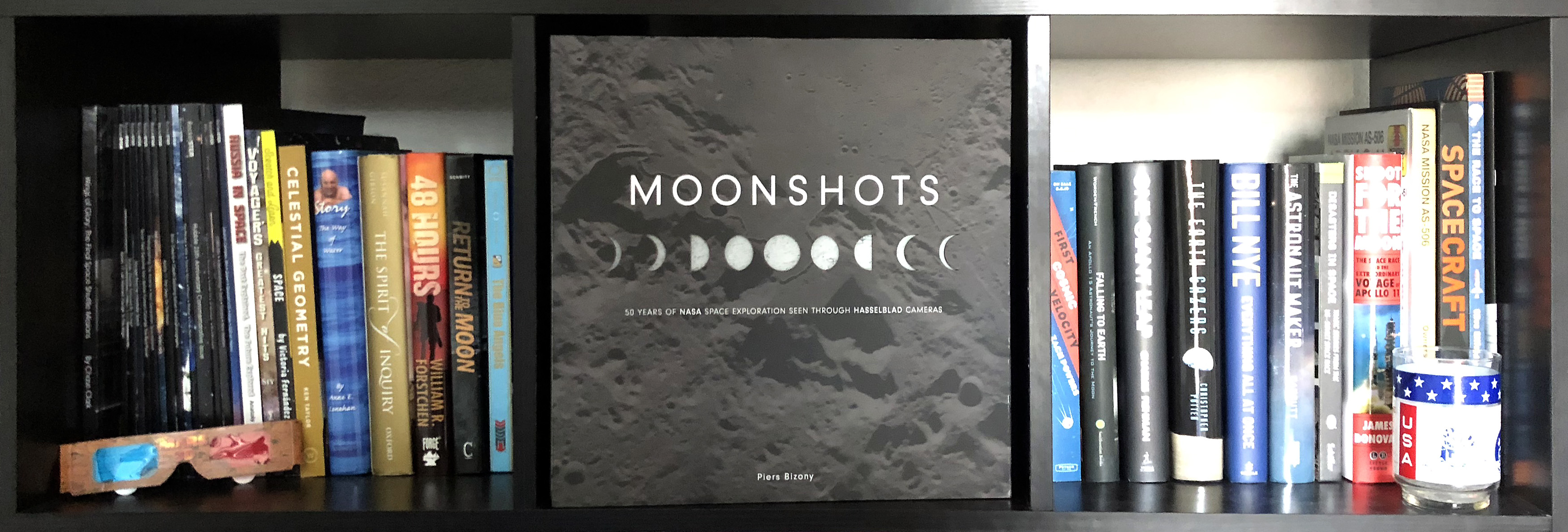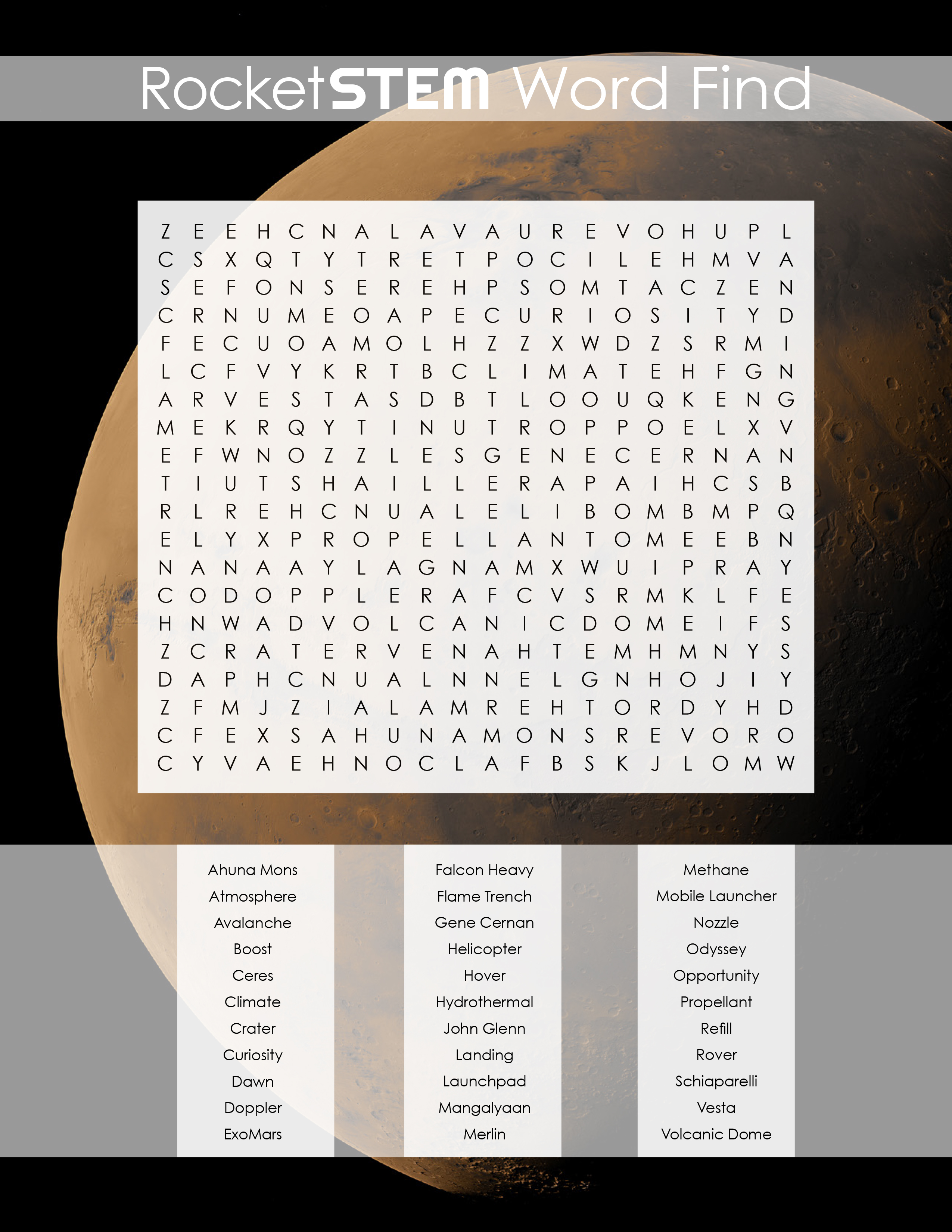# Exploring the surface of a comet

On August 6, 2014 after a decade-long journey chasing its target, ESA’s Rosetta has today become the first spacecraft to rendezvous with a comet, opening a new chapter in Solar System exploration. Comet 67P/Churyumov–Gerasimenko and Rosetta now lie 405 million kilometers from Earth, about half way between the orbits of Jupiter and Mars, rushing towards the inner Solar System at nearly 55,000 kilometers per hour.

This image shows the comet close-up from a distance of 283 kilometers. The image has a width of 5 kilometers.

Problem 1 – Approximate the shape of the dumbbell-shaped nucleus as two spheres. What is the total volume of the nucleus in cubic meters?

Problem 2 – If the average density of the material is 300 kg/m3, about what is the total mass of the nucleus in metric tons?

Problem 1 – Approximate the shape of the dumbbell-shaped nucleus as two spheres. What is the total volume of the nucleus in cubic meters?Answer: The image shows one way to draw two spheres inside the nucleus to represent the volume. From the scale of the image the larger sphere has a radius of about 1200 meters and the smaller sphere has a radius of about 840 meters. From the volume of a sphere V = 4/3 π R3, we have:

Large sphere = 1.3 x 3.1 x (1200)3

= 7.0 x 109 cubic meters

Small sphere = 1.3 x 3.1 x (840)

= 2.4 x 109 cubic meters.

Problem 2 – If the average density of the material is 300 kg/m3, about what is the total mass of the nucleus in metric tons?

Answer: The total volume is just 7.0 x 109 + 2.4 x 109 = 9.4 x 109 cubic meters.
From Mass = density x volume we then get:

= 300 kg/m3 x 9.4×109 m3

= 2.8 x 1012 kg

Since 1 ton = 1000 kg, the mass is about 2.8 billion tons!

SpaceMath@NASA introduces students to the use of mathematics in today’s scientific discoveries. Through press releases and other articles, it explores how many kinds of mathematics skills come together in exploring the universe.

Visit http://spacemath.gsfc.nasa.gov to discover hundreds of math problems you can solve on your own.